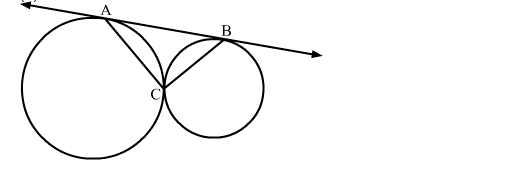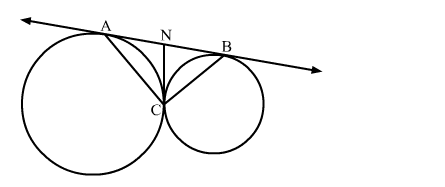# In the given figure, two circles touch each other at C and AB is a tangent to both the circles.`
Question:

In the given figure, two circles touch each other at C and AB is a tangent to both the circles. The measure of ∠ACB is
(a) 45
(b) 60
(c) 90
(d) 120Solution:We know that tangent segments to a circle from the same external point are congruent.
Therefore, we have
NA = NC and NC = NB
We also know that angle opposite to equal sides are equal
∴ ∠NAC = ∠NCA and ∠NBC = ∠NCB
Now, ∠ANC + ∠BNC = 180           [Linear pair angles]
⇒ ∠NBC + ∠NCB + ∠NAC + ∠NCA= 180      [Exterior angle property]
⇒ 2∠NCB + 2∠NCA= 180
⇒ 2(∠NCB + ∠NCA) = 180
⇒ ∠ACB = 90
Hence, the correct answer is option (c).